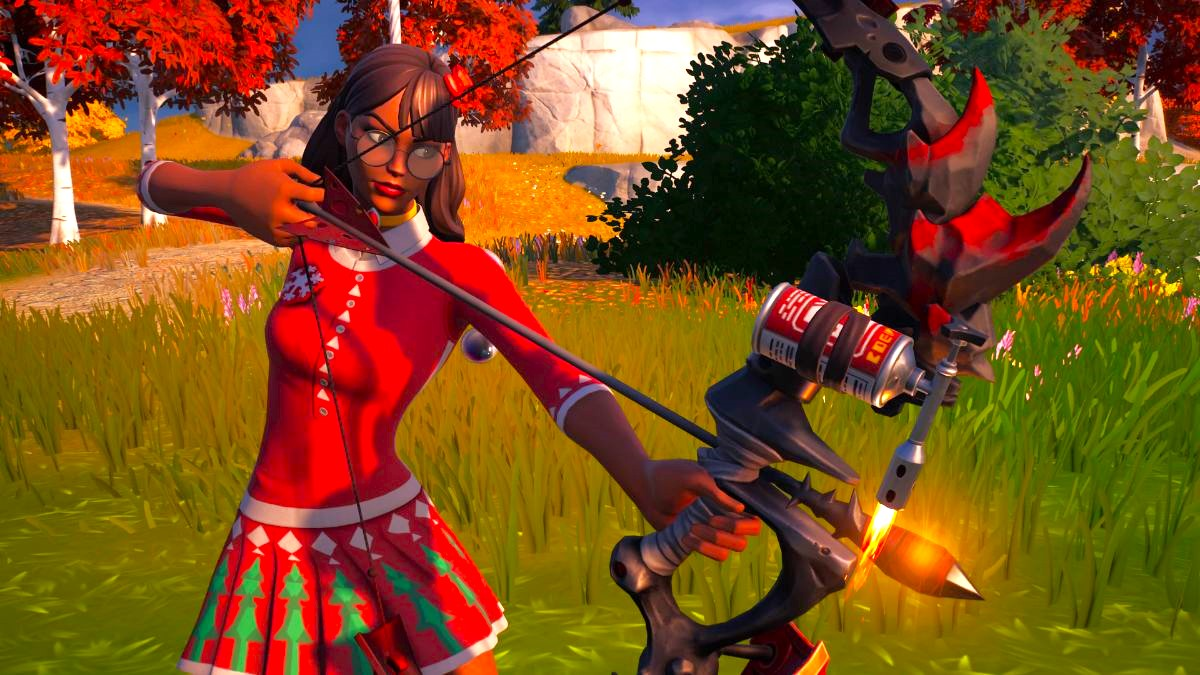# How to solve and decode encrypted Cipher quests in Fortnite

Cipher quests prove to be the toughest Fortnite challenges yet.Screenshot by Gamepur

As Chapter 4 Season 1 comes to an end, Fortnite is tasking players with not only completing Cipher challenges but the puzzles inside each one. Rather than giving a description for each challenge, its “encrypted” quests only list a series of numbers, and you must solve for their letters in order to make sense of your objective. This guide will examine what encrypted Cipher quests are in Fortnite and how they can be solved.

## How to do the encrypted Cipher quests in Fortnite

Until March 7, a new encrypted Cipher quest can be completed in the battle royale, though each one is expected to have a different code. For instance, the first of these challenges is only listed as follows: “1.17.23.9.14. 19.19.24.1.21.6.” At the time of writing, it is not confirmed how these numbers can be translated, as matching each letter to their respective number in the alphabet (such as 1 = A, 2 = B, and so forth) only results in nonsense.

However, it appears players must subtract each number from another code given out by Fortnite’s Twitter before the challenges went live: “0.3.1.0.2.0.2.3.” Once that is done, the outcome of each equation should be translated to its respective letter. By doing so, the method coincidentally spells out a named location on the map. As shown below, you can use this method on the first encrypted challenge to spell out Anvil Square.

• 1 – 0 = 1, 1 = A
• 17- 3 = 14, 14 = N
• 23 – 1 = 22, 22 = V
• 9 – 0 = 9, 9 = I
• 14 – 2 = 12, 12 = L
• 19 – 0 = 19, 19 = S
• 19 – 2 = 17, 17 = Q
• 24 – 3 = 21, 21 = U
• 1 – 0 = 1, 1 = A
• 21 -3 = 18, 18 = R
• 6 -1 = 5, 5 = E

With the location solved, players should then take a peek at the quest’s subtext. For the first encrypted Cipher challenge, it hints that you must “Inspect the wall… beneath the eastern building at…,” and this equation fittingly fills in the rest. Aside from Cipher challenges, players will also need to do some deciphering in the Oathbound questline, with its first objective having you decipher the Rift Gate’s coordinates.